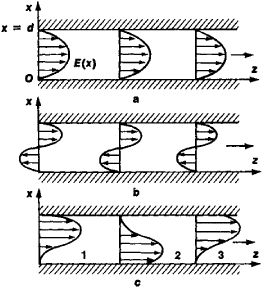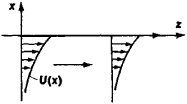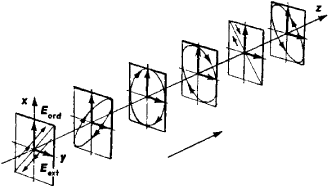# Normal Waves

The following article is from The Great Soviet Encyclopedia (1979). It might be outdated or ideologically biased.

## Normal Waves

harmonic waves of a particular physical nature (electromagnetic, elastic, and so on) that preserve the transverse structure of the field and/or polarization during rectilinear propagation. In this respect they differ from all other waves that are capable of propagating in a given system. For example, when electromagnetic normal waves propagate between parallel metal plates (Figure 1), the transverse structure of their electric field (with respect to the direction of propagation) is identical in all cross sections. However, the transverse structure of all other waves, which differ from normal waves, is not preserved during propagation. Thus, the wave form resulting from the superposition of the two normal waves depicted in Figure 1,a and 1,b changes from section to section (Figure 1,c).Figure 1. Propagation of two normal waves (a) and (b) and the wave (c) produced by their superposition. In cross sections (1) and (3) the phase difference of the normal waves is ϕ = 0, and they are added, but in cross section (2) ϕ = –π, and the second wave is subtracted.

Electromagnetic normal waves in wave-guide systems used to transmit information or electromagnetic energy are of the most practical interest. Such systems include superhigh-frequency (SHF) radio wave guides, coaxial cables, plasma wave guides, ionospheric and tropospheric long-distance radio communications channels, light guides made of glass fibers, and “quasi-optical” transmission lines for millimeter and submillimeter waves.

Normal waves find important applications in acoustic waveguide systems (acoustic pipes; sound channels in the ocean and the troposphere); elastic normal waves are significant in plates (Lamb waves and “transverse” normal waves) and rods (longitudinal, flexural, and torsional normal waves). In particular, elastic normal waves are used in ultrasonic delay lines and in determining elastic and other parameters of solid bodies.

The number N of normal waves that can propagate in the systems mentioned above depends on the ratio of the wavelength λ and the transverse dimensions d of the system. For waves of a given frequency, this number is always finite, and the value of N increases with an increase in the ratio d/λ. At very low frequencies (that is, when d/λ ≪ ½), only one normal wave of a given type can propagate, and in some systems, such as hollow radio-frequency wave guides, the propagation of low-frequency normal waves is absolutely impossible. The phase and group velocities of the different types of normal waves are different (this, in particular, explains the distortion of the transverse field structure when several normal waves are superposed, as in Figure 1). Therefore, it is desirable to use only one type of normal wave in the transmission of information.

Normal waves are of physical significance because any disturbance in a region free of sources may be represented as the superposition of normal waves, and the resultant elastic or electromagnetic energy flow is equal to the sum of the flows in all the normal waves. In this respect the concept of normal waves plays a role in wave theory analogous to the concept of normal vibrations in the theory of vibrating systems.

Surface normal waves may propagate along the interface between two media. Examples are Rayleigh waves on the boundary of an elastic body (Figure 2) and slow electromagnetic waves in slow-wave structures. In the case of normal waves in multiwire coupled transmission lines, which are used in communications technology, the ratio of the oscillation amplitudes in the separate conductors, rather than the transverse field distribution, is preserved along the direction of propagation.

Finally, normal waves in infinite and homogeneous continuous media are plane waves, which preserve their polarizationFigure 2. Propagation of a Ray-leigh wave on the boundary of an elastic body

during propagation. For example, both the ordinary and extraordinary waves in uniaxial crystals are normal waves. The waves are linearly polarized in mutually perpendicular directions, and their polarizations in the direction of propagation are preserved (Figure 3), whereas the polarization of an arbitrarily polarized wave varies from point to point. Other examples of normal waves in continuous media are plane elastic waves, elliptically polarized electromagnetic waves in a magnetic plasma, and circularly polarized waves in optically active media.Figure 3. Propagation of ordinary and extraordinary waves in uniaxial crystals

### REFERENCES

Gorelik, G. S. Kolebaniia i volny, 2nd ed. Moscow, 1959.
Brillouin, L., and M. Parodi. Rasprostranenie voln v periodicheskikh strukturakh. Moscow, 1959. (Translated from French.)
Brekhovskikh, L. M. Volny v sloistykh sredakh. Moscow, 1973.
Vainshtein, L. A. Elektromagnitnye volny. Moscow, 1957.
Bergmann, L. Ul’trazvuk i ego primenenie v nauke i tekhnike. Moscow, 1956. (Translated from German.)
Viktorov, I. A. Fizicheskie osnovy primeneniia ul’trazvukovykh voln Releia i Lemba v tekhnike. Moscow, 1966.

IU. A. KRAVTSOV

References in periodicals archive ?
There was damage at 100ft above sea level on the platform, so you have the Atlantic running mad for several days and pretty good anecdotal evidence that there are freak waves two or three times the height of normal waves.
It is generally known that in a lossless MCML consisting of N conductors, N normal modes (or normal waves) can be propagated (Tripathi, Lee 1989).
"In such structures, electromagnetic waves are coupled to electron plasma in the metal, which gives rise to their special name--plasmons." Unlike normal waves in dielectric media, such as air, plasmons can be focused or concentrated into spatial regions that are much smaller than the wavelength (which is called sub-wavelength localisation).
The combination of storm-surge caused coastal flooding and larger than normal waves can quickly devastate an area.
As normal waves propagate, they spread out according to the laws of diffractions.
It is known, that, generally, in a lossless MMCL consisting of (N - 1) conductors can propagate N normal waves (or normal modes), which are characterized by N propagation factors [[beta].sub.t] and [N.sup.2] characteristic impedances [Z.sub.ki] (where k is type of normal mode; i is conductor number) .
Phase and group velocities of different types of normal waves differ from each other in the MMCL, as a result the interference of the propagated normal waves occurs in the MMCL and transmitted signals suffer from distortions.
By contrast, the normal waves you see at the beach, which are caused by wind and tides, travel relatively slowly and are only one to two meter's deep.
For flooding to occur on open coastlines, an extra factor is needed to add to the height of the sea surface in addition to normal waves and tides, and severe meteorological conditions can provide this in the form of a sea surge.
It is known, that, generally, in an MMCL consisting of N conductors (plus ground plane), N normal waves (or normal modes) can propagate .
Modelling various microwave devices by the multiconductor line approach it is enough to confine to two main normal waves only .

Site: Follow: Share:
Open / Close